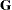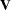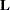The GLIMMIX Procedure
 ODS Table Names

Each table created by PROC GLIMMIX has a name associated with it, and you must use this name to reference the table when you use ODS statements. These names are listed in Table 38.20.

Table 38.20 ODS Tables Produced by PROC GLIMMIX

Table Name

Description

Required Statement / Option

AsyCorr

asymptotic correlation matrix of covariance parameters

PROC GLIMMIX ASYCORR

AsyCov

asymptotic covariance matrix of covariance parameters

PROC GLIMMIX ASYCOV

CholG

Cholesky root of the estimatedmatrix

CholV

Cholesky root of blocks of the estimatedmatrix

ClassLevels

level information from the CLASS statement

default output

Coefmatrix coefficients

E option in MODEL, CONTRAST, ESTIMATE, LSMESTIMATE, or LSMEANS; ELSM option in LSMESTIMATE

ColumnNames

name association for OUTDESIGN data set

PROC GLIMMIX
OUTDESIGN(NAMES)

CondFitStatistics

conditional fit statistics

PROC GLIMMIX
METHOD=LAPLACE

Contrasts

results from the CONTRAST statements

ConvergenceStatus

status of optimization at conclusion

default output

CorrB

approximate correlation matrix of fixed-effects parameter estimates

CovB

approximate covariance matrix of fixed-effects parameter estimates

CovBDetails

MODEL / COVB(DETAILS)

CovBI

inverse of approximate covariance matrix of fixed-effects parameter estimates

CovBModelBased

model-based (unadjusted) covariance matrix of fixed effects if DDFM=KR or EMPIRICAL option is used

MODEL / COVB(DETAILS)

CovParms

estimated covariance parameters in GLMMs

default output (in GLMMs)

CovTests

results from COVTEST statements (except for confidence bounds)

Diffs

differences of LS-means

LSMEANS / DIFF (or PDIFF)

Dimensions

dimensions of the model

default output

Estimates

results from ESTIMATE statements

FitStatistics

fit statistics

default

G

estimatedmatrix

GCorr

correlation matrix from the estimatedmatrix

Hessian

Hessian matrix (observed or expected)

PROC GLIMMIX HESSIAN

InvCholG

inverse Cholesky root of the estimatedmatrix

InvCholV

inverse Cholesky root of the blocks of the estimatedmatrix

InvG

inverse of the estimatedmatrix

InvV

inverse of blocks of the estimatedmatrix

IterHistory

iteration history

default output

kdTree

k-d tree information

RANDOM / TYPE=RSMOOTH KNOTMETHOD= KDTREE(TREEINFO)

KnotInfo

knot coordinates of low-rank spline smoother

LSMeans

LS-means

LSMEstimates

estimates among LS-means

LSMFtest

F test for LSMESTIMATEs

LSMLines

lines display for LS-means

ModelInfo

model information

default output

NObs

number of observations read and used, number of trials and events

default output

OddsRatios

odds ratios of parameter estimates

OptInfo

optimization information

default output

ParameterEstimates

fixed-effects solution; overdispersion and scale parameter in GLMs

ParmSearch

parameter search values

ResponseProfile

response categories and category modeled

default output in models with binary or nominal response

Slices

tests of LS-means slices

SliceDiffs

differences of simple LS-means effects

SolutionR

random-effects solution vector

StandardizedCoefficients

fixed-effects solutions from centered and/or scaled model

Tests1

Type I tests of fixed effects

Tests2

Type II tests of fixed effects

Tests3

Type III tests of fixed effects

default output

V

blocks of the estimatedmatrix

VCorr

correlation matrix from the blocks of the estimatedmatrix

The SLICE statement also creates tables, which are not listed in Table 38.20. For information about these tables, see the section SLICE Statement of Chapter 19, Shared Concepts and Topics.Previous Page | Next Page | Top of Page